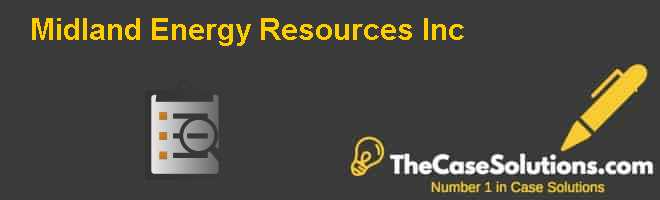Midland Energy Resources Inc. Harvard Case Solution & AnalysisCost of Equity

Cost of equity is quite a complex calculation and we have two famous models for the calculation of cost of equity out of which more reasonable and well-known model is Capital Asset Pricing Model (CAPM) and the calculation of this model is performed by the following formula,

However, the CAPM model uses different variable as shown in the formula above,

 Cost of Equity Variables Description Re Cost of equity Rf Risk free rate Β Equity beta EMRP Equity market risk premium

Risk Free Rate

Risk free rate as its name suggests that this is the rate at which the investment will not be exposed to any risk at all, meanwhile, such a rate in practice does not exist and even the government’s Treasury bill contains risk but since they are the most low risk investments; hence, it is considered to be risk free rates. However, Midland is provided with the rates on the US treasury bills for the period of 1 year, 10 years and 30 years and most relevant rate will be the one with similar duration; therefore, the most reasonable rate will be 4.66% on 10 years’ treasury bond.

Beta

Beta is the measure of systematic risk of industry and in other words it measures the sensitivity of a stock’s returns to market returns. Technically, it is measured as a co-variance of stock returns with market returns divided by the variance of stock. However, an alternative approach, as used by Midland is to calculate beta by looking at outside sources like competitors’ betas and analysts’ reports. Midland has come up with a company beta of 1.33 using outside information. However, the betas for internal divisions of Midland are hard to calculate. A number of competitor betas for Exploration & Production and Refining & Marketing divisions have been provided. An average of the betas for competitors has been assumed as appropriate betas for the respective two divisions of Midland. However, in the case of Refining & Marketing, the Petrarch Fuel Services has been omitted when calculating the average beta. Petrarch Fuel Services does not appear to be similar to Midland. Apart from its unusual leverage and beta, the company has very low long-term revenue and earnings. It is considered that the company may significantly differ from Midland in terms of size and operations.

A major hurdle arises in estimating the beta for the Petrochemicals division. There are no comparable Petrochemicals firms within the industry. Therefore, the industry average cannot be used to calculate the relevant beta. Similarly, historical data for Midland is not available as stock returns cannot be bifurcated between the different divisions. A possible approach is to distribute the overall beta of Midland among the three divisions on the basis of weighted asset values. It can be assumed that the overall beta of Midland is the asset weighted average of the betas of the individual divisions:

Based on the asset weights for the year end 2006 and the betas obtained from industry data, we find the missing beta for Petrochemicals to be 1.09. The technique of obtaining the beta for the Petrochemicals is not very robust. It is strictly based on the assertion that the asset weighted average betas of each division constitutes the overall beta of the company. This may not be a very far-off contention if the betas of other divisions and the company beta are accurately calculated. However, the fact is that the estimates of the other betas are also speculative in nature. This makes the beta estimate for the Petrochemicals division highly subjective. However, it appears to be the only way to calculate the beta with the available information, and we have no choice but to employ it in the calculations.

Equity Market Risk Premium (EMRP)

The Equity Market Risk Premium (EMRP) is the premium awarded for taking systematic risk. Systematic risk is the risk of the overall market. The premium is usually measured as the difference between market return and risk free return. In this case study, the US Treasury bond returns have been taken as a measure of risk free return. However, the risk free return can be taken to be some other return as well. For instance, the 1 year US treasury bills interest serves as a good measure of the short-term risk free return................................

This is just a sample partial case solution. Please place the order on the website to order your own originally done case solution.Other Similar Case Solutions like